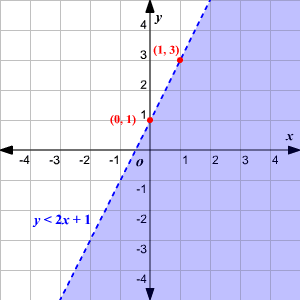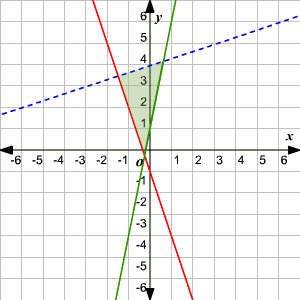# Systems of Linear Inequalities

One linear inequality in two variables divides the plane into two half-planes . To graph the inequality, graph the equation of the boundary.  Use a solid line if the symbol $\le$ or $\ge$ is used because the boundary is included in the solution.  Use a dashed line if $<\text{\hspace{0.17em}}\text{\hspace{0.17em}}\text{or}\text{\hspace{0.17em}}\text{\hspace{0.17em}}>$ is used to indicate that the boundary is not part of the solution. Shade the appropriate region. Unless you are graphing a vertical line the sign of the inequality will let you know which half-plane to shade.  If the symbol $\ge$ or $>$ is used, shade above the line.  If the symbol $\le$ or $<$ is used shade below the line. For a vertical line, larger solutions are to the right and smaller solutions are to the left. A system of two or more linear inequalities can divide the plane into more complex shapes.

Example 1:

Graph $y<2x+1$Example 2:

Graph the system of linear inequalities.

${y}{<}\frac{{1}}{{3}}{x}{+}{4}$

${y}{\ge }{-}{3}{x}{-}{1}$

${y}{\ge }{5}{x}{+}{1}$

Graphing the three lines and shading the region enclosed, we get the figure below.